pandas python 分离_python数据存储系列教程—python(pandas) - CSDN

• ## python(pandas模块)

千次阅读 2019-02-27 16:15:50
1.什么是pandas？ numpy模块和pandas模块都是用于处理数据的模块。 numpy主要用于针对数组进行统计计算，处理数字数据比较方便。 pandas除了可以处理数字数据，还可以处理字符串数据，再其模块中包含两种数据类型 ...
1.什么是pandas？

numpy模块和pandas模块都是用于处理数据的模块。
numpy主要用于针对数组进行统计计算，处理数字数据比较方便。
pandas除了可以处理数字数据，还可以处理字符串数据，再其模块中包含两种数据类型

一维数据类型：Series
二维: DataFrame
三维: Panel  …
四维: Panel4D  …
N维: PanelND  …
最常用的数据类型是一维和二维

pandas创建Series数据类型
import pandas as pd
# 查看pandas的版本号
print(pd.__version__)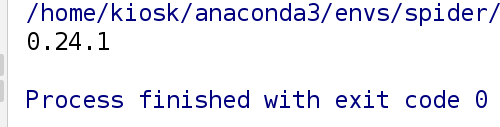1. 通过列表创建Series对象
# 通常将pandas模块名起作pd
import pandas as pd
li=['包子','馒头','煎饼']
# 不指定索引，行号默认从0开始
data1 = pd.Series(data=li)
print(data1)
# 指定索引
data2=pd.Series(data=li,index=["A","B","C"])
print(data2)
print(type(data2))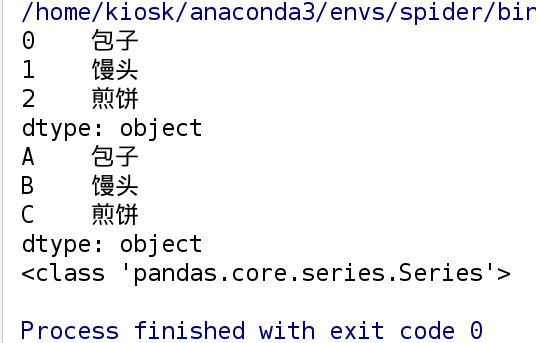2. 通过numpy的对象Ndarray创建Series
import pandas as pd
import numpy as np
# 随机生成5个小数
arry=np.random.randn(5)
data=pd.Series(data=arry)
print(data)
# 修改元素的数据类型
print(data.astype(np.int))
# 修改元素保留三位小数
print(data.round(3))
print(type(data))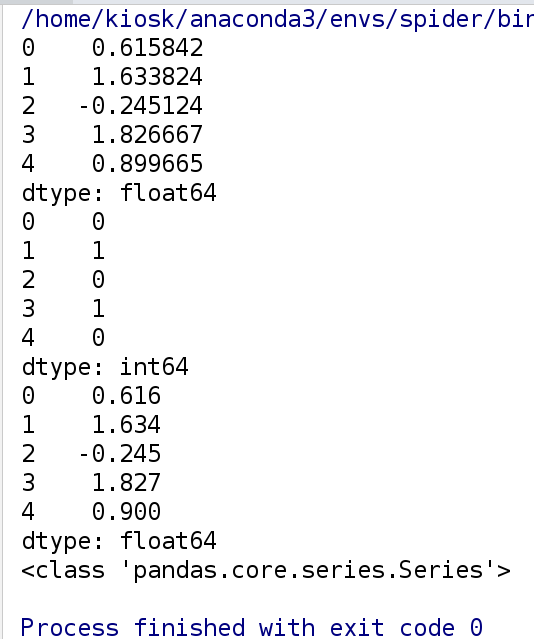3. 通过字典创建Series对象
import string
import pandas as pd
dict = {string.ascii_lowercase[i]:i for i in range(10)}
# 数据的行号为字典的key值，数据的元素为字典的value值
s3 = pd.Series(dict)
print(s3)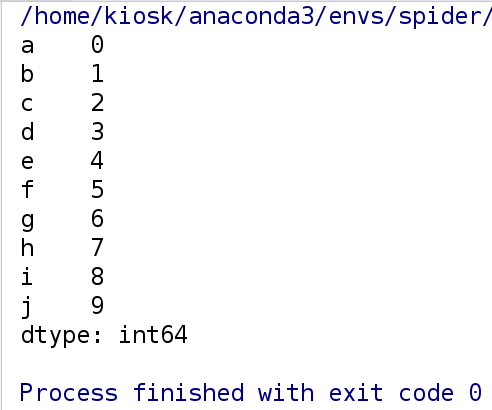Series基本操作
1. 修改Series索引
import pandas as pd
import numpy as np
import  string
array = ["粉条", "粉丝", "粉带"]
s1 = pd.Series(data=array)
print(s1)
# 修改Series索引
s1.index=['A','B','C']
print(s1)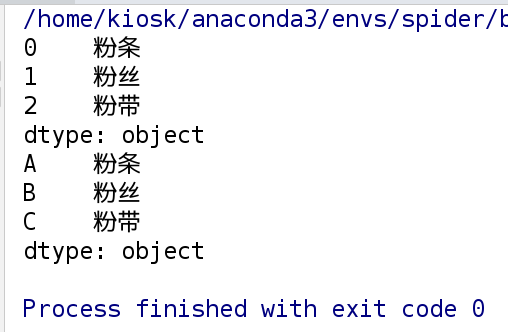2. Series纵向拼接
array = ["粉条", "粉丝", "粉带"]
s2 = pd.Series(data=array)
# Series竖直拼接
s3=s1.append(s2)
print(s3)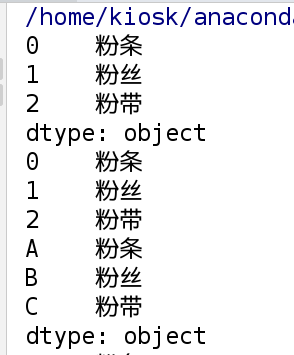3. 删除指定索引对应的元素
# 3). 删除指定索引对应的元素;
s3 = s3.drop('C')  # 删除索引为‘C’对应的值;
print(s3)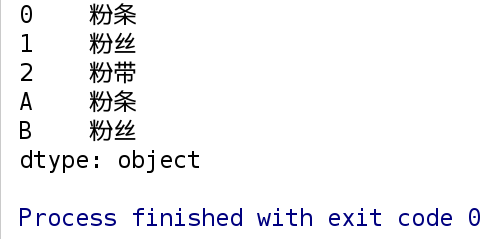4. 根据指定的索引查找元素
print(s3['B'])
# 根据指定索引修改元素
s3['B'] = np.nan
print(s3)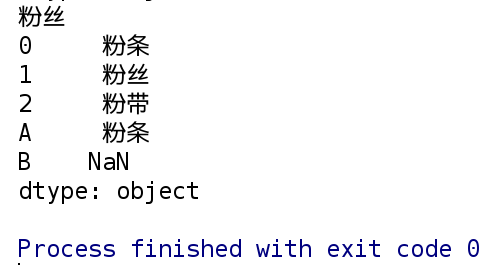5. 切片操作  — 同列表
print(s3[:2])   # 显示前两个元素
print(s3[::-1]) # 显示除最后一个元素外的所有元素
print(s3[-2:])  # 显示最后两个元素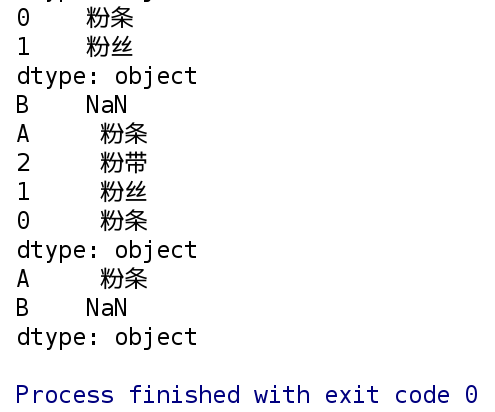Series的基本运算
按照对应的索引进行计算， 如果索引不同，则填充为Nan
import pandas as pd
import numpy as np
import  string
# 创建两个Series对象
s1  = pd.Series(np.arange(5), index=list(string.ascii_lowercase[:5]))
s2  = pd.Series(np.arange(2, 8), index=list(string.ascii_lowercase[2:8]))

print(s1)
print(s2)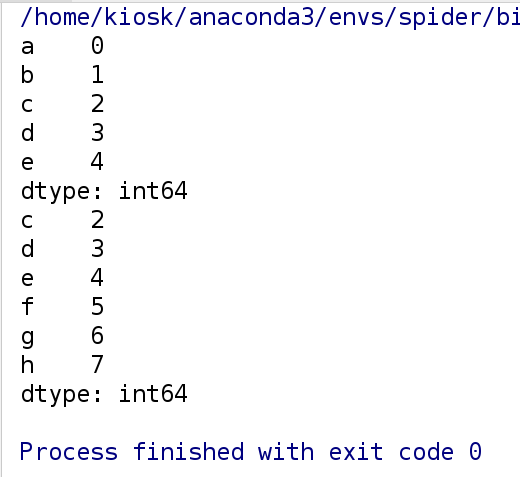# 加法
print(s1 + s2)

# 减法
print(s1 - s2)
print(s1.sub(s2))

# 乘法
print(s1 * s2)
print(s1.mul(s2))

# 除法
print(s1 / s2)
print(s1.div(s2))

# 求中位数
print(s1)
print(s1.median())

# 求和
print(s1.sum())

# 求最大值
print(s1.max())

# 求最小值
print(s1.min())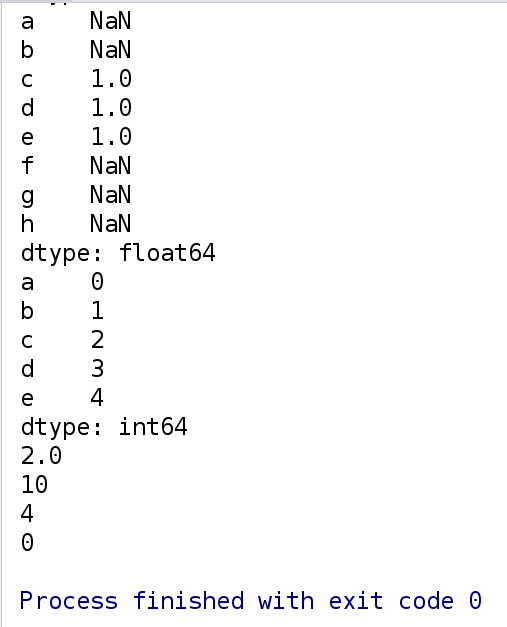特殊的where方法
series中的where方法运行结果和numpy中完全不同

numpy中的where方法可以用来查找数据中符合不等式的索引，也可以将数据中符合不等式的元素重新赋值
pandas的series中的where方法只能将数据中符合不等式的元素重新赋值

import pandas as pd
import numpy as np
import  string

s1 = pd.Series(np.arange(5), index=list(string.ascii_lowercase[:5]))
# s1中小于3的元素赋值为缺失值
print(s1.where(s1 > 3))

# 对象中小于3的元素赋值为10；
print(s1.where(s1 > 3, 10))

# 对象中大于3的元素赋值为10；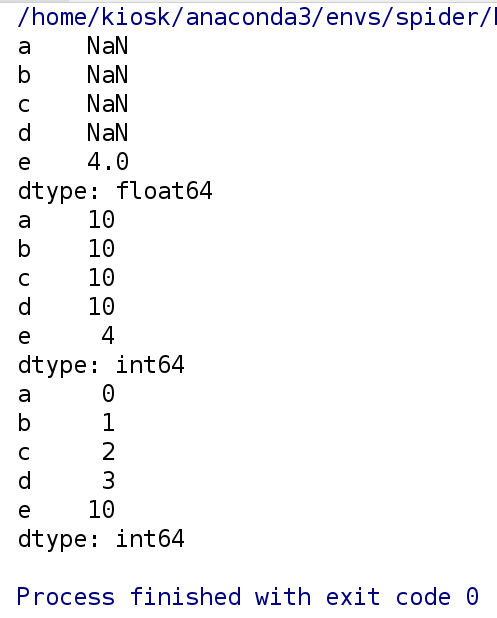创建DataFrame数据类型
方法1： 通过列表创建
import pandas as pd
li = [
[1, 2, 3, 4],
[2, 3, 4, 5]
]

# DataFRame对象里面包含两个索引， 行索引(0轴， axis=0)， 列索引(1轴， axis=1)
d1 = pd.DataFrame(data=li, index=['A', 'B'], columns=['views', 'loves', 'comments', 'tranfers'])
print(d1)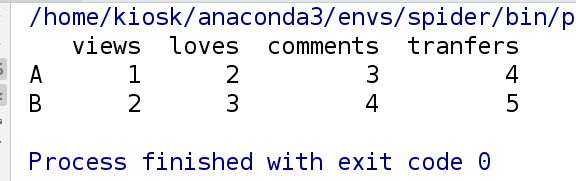方法2： 通过numpy对象创建
import pandas as pd
import numpy as np
narr = np.arange(8).reshape(2, 4)
# DataFRame对象里面包含两个索引， 行索引(0轴， axis=0)， 列索引(1轴， axis=1)
d2 = pd.DataFrame(data=narr, index=['A', 'B'], columns=['views', 'loves', 'comments', 'tranfers'])
print(d2)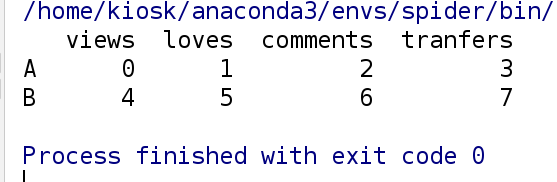方法三: 通过字典的方式创建
import pandas as pd
# 字典中的key值为数据中的列索引
# 字典中的value值为数据中的元素
dict = {
'views': [1, 2, ],
'loves': [2, 3, ],

}
# index中的行索引必须和字典中key-value值个数相对应
d3 = pd.DataFrame(data=dict, index=['粉条', "粉丝"])
print(d3)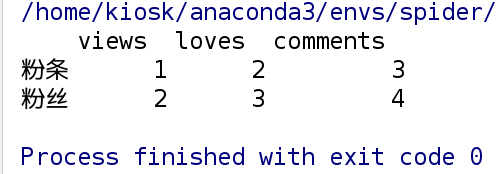4.日期操作的特例:
# date_range() ：生成日期索引值对象的函数
# start表示起始日期；end表示截至日期；
# freq表示按xx间隔将起始到截至日期分隔开（默认以一天为间隔）；periods表示xx个分组
dates = pd.date_range(start='1/1/2018', end='1/08/2018')
print(dates)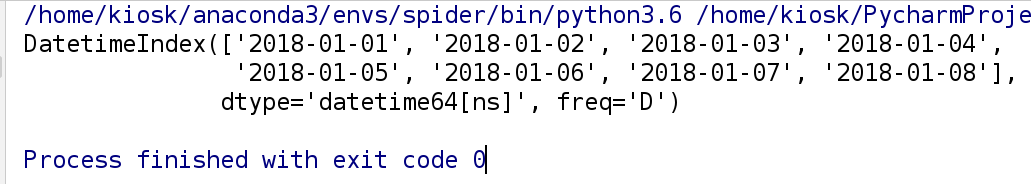# 行索引
dates = pd.date_range(start='today', periods=6)
# 数据
data_arr = np.random.randn(6, 4)
# 列索引
columns = ['A', 'B', 'C', 'D']
d4 = pd.DataFrame(data_arr, index=dates, columns=columns)
print(d4)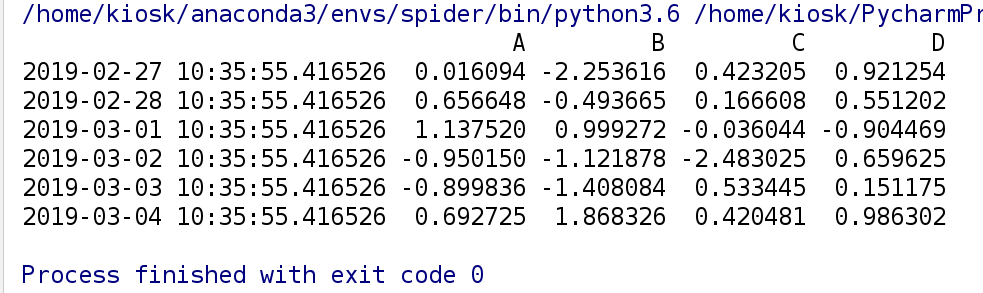练习：
一维对象: 建立一个以2019年每一天作为索引， 值为随机数；
import pandas as pd
import numpy as np

dates=pd.date_range(start='1/01/2019',end='12/31/2019',freq='D')
data=np.random.randn(len(dates))
s=pd.Series(data=data,index=dates)
# 查看前5行
# 查看后5行
print(s.tail())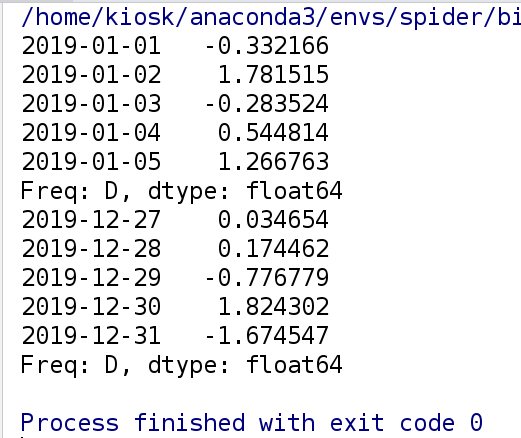DataFrame的基本操作
# 先创建一个DataFrame对象用于测试
import pandas as pd
import numpy as np

narr = np.arange(8).reshape(2, 4)
# DataFRame对象里面包含两个索引， 行索引(0轴， axis=0)， 列索引(1轴， axis=1)
d2 = pd.DataFrame(data=narr, index=['A', 'B'], columns=['views', 'loves', 'comments', 'tranfers'])
print(d2)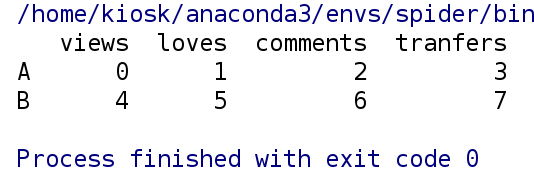1. 查看基础属性
print(d2.shape)  # 获取行数和列数;
print('*'*30)
print(d2.dtypes)  # 列数据类型
print('*'*30)
print(d2.ndim)  # 获取数据的维度
print('*'*30)
print(d2.index) # 行索引
print('*'*30)
print(d2.columns) # 列索引
print('*'*30)
print(d2.values, type(d2.values))   # 对象的值， 二维ndarray数组;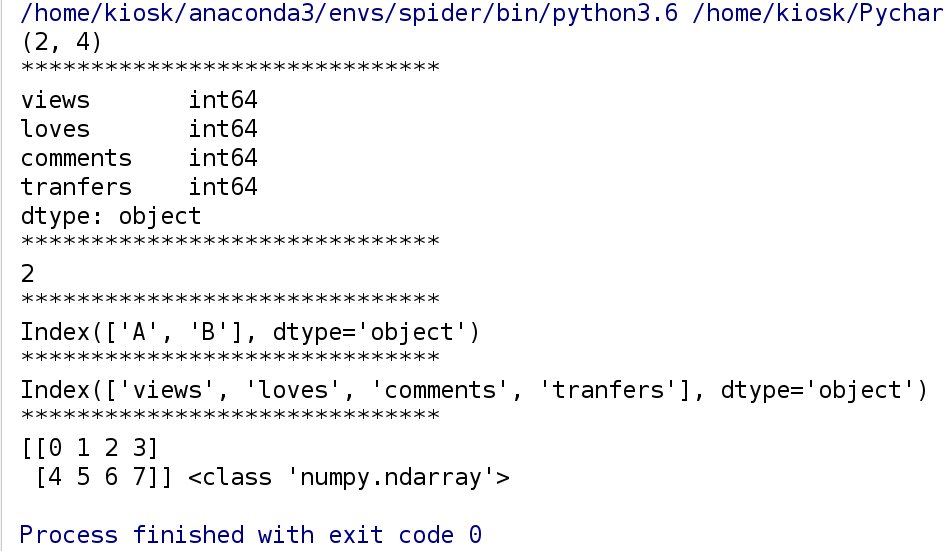2. 数据整体状况的查询
# 相关信息的预览： 行数， 列数， 列类型， 内存占用
print("info:", d2.info())

print("统计".center(50, '*'))
# 快速综合用计结果： 计数， 均值， 标准差， 最小值， 1/4位数， 中位数， 3/4位数， 最大值;
print(d2.describe())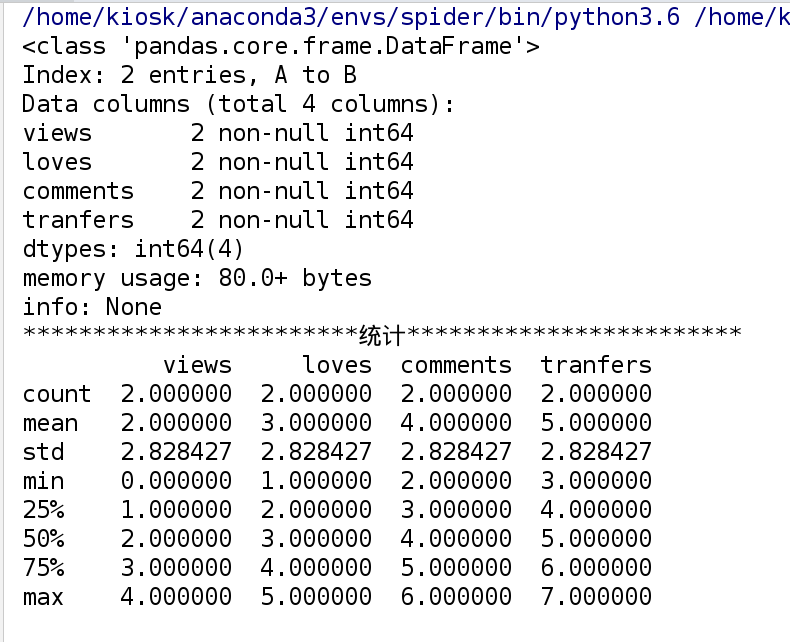3.转置及按列排序
# 3). 转置操作
print(d2.T)
print('*'*30)
# 4). 按列进行排序
print(d2)
# 按照指定列进行排序， 默认是升序， 如果需要降序显示，设置ascending=False;
print(d2.sort_values(by="views", ascending=False))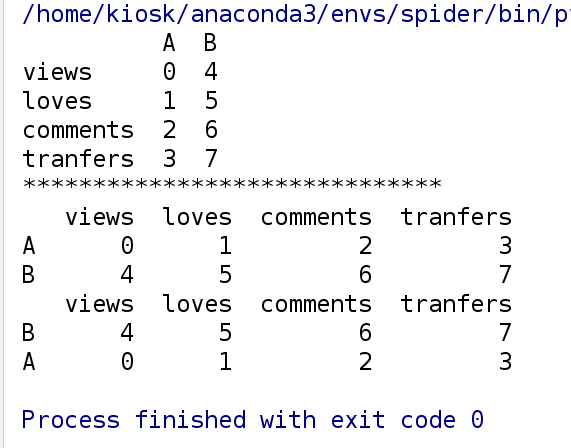4.切片及查询
print(d2[:1])   # 可以实现切片， 但是不能索引;
print('*'*30)
print('1:\n', d2['views'])   # 通过标签查询， 获取单列信息
print('2:\n', d2.views)   # 和上面是等价的;
print('*'*30)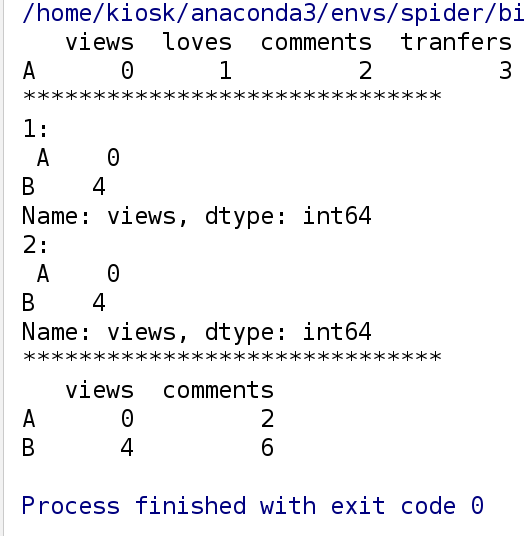5.通过类似索引的方式查询

iloc(通过位置进行行数据的获取),
loc(t通过标签索引行数据)

print(d2)
print('第一行元素：\n')
# iloc查询
print(d2.iloc)
# loc查询
print(d2.loc['A'])
print('第二行元素：\n')
# iloc查询
print(d2.iloc[-1:])
# loc查询
print(d2.loc['B'])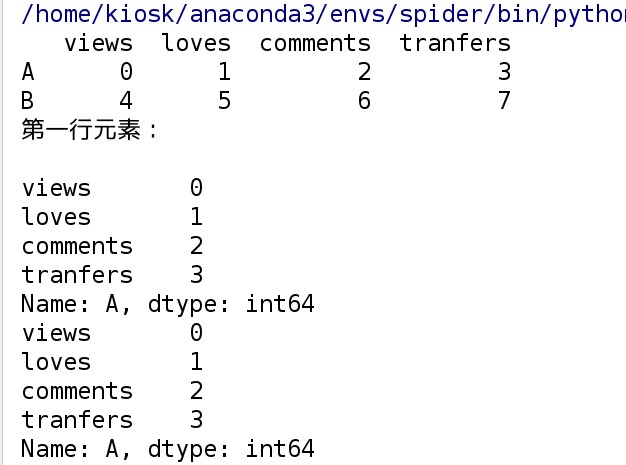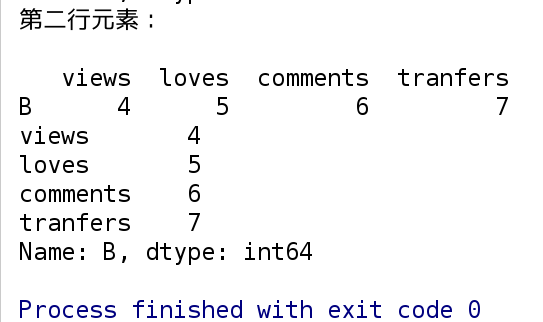6. 更改pandas的值
类似于列表根据索引修改值，通过iloc或loc方法进行修改pandas的值
print(d2)
d2.loc['A'] = np.nan
print(d2)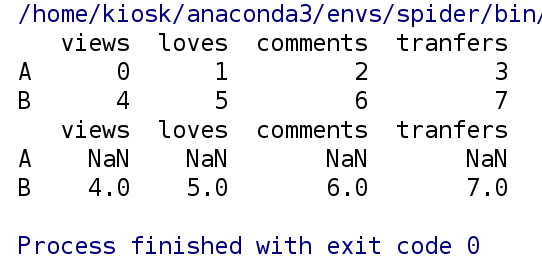从文件中读取数据
1. csv文件的写入
df = pd.DataFrame(
{'province': ['陕西', '陕西', '四川', '四川', '陕西'],
'city': ['咸阳', '宝鸡', '成都', '成都', '宝鸡'],
'count1': [1, 2, 3, 4, 5],
'count2': [1, 2, 33, 4, 5]
}
)

df.to_csv('doc/csvFile.csv')
print("csv文件保存成功")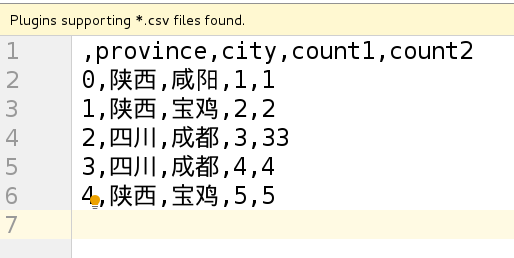2. csv文件的读取
# 在读取的过程中，会将csv文件中行索引读取出来，另起一列Unnamed: 0
print(df2)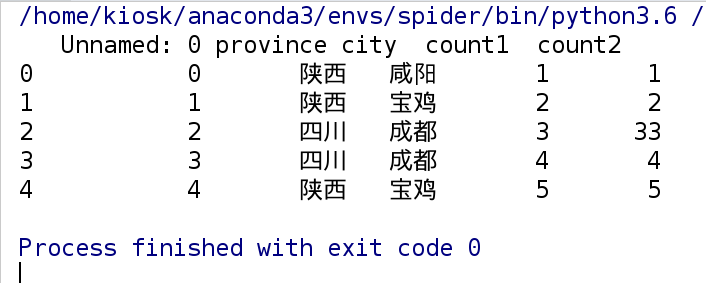3. excel文件的写入
df2.pop('Unnamed: 0')
df2.to_excel("/tmp/excelFile.xlsx", sheet_name="省份统计")
print("excel文件保存成功")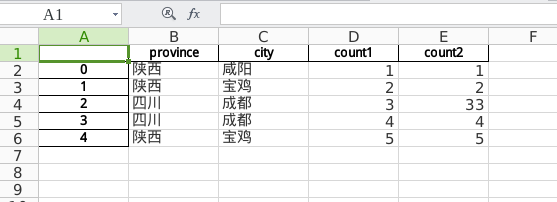分组与聚合操作之groupby
pandas提供了一个灵活高效的groupby功能，

它使你能以一种自然的方式对数据集进行切片、切块、摘要等操作。
根据一个或多个键（可以是函数、数组或DataFrame列>名）拆分pandas对象。
计算分组摘要统计，如计数、平均值、标准差，或用户自定义函数。

import pandas as pd

df = pd.DataFrame(
{'province': ['陕西', '陕西', '四川', '四川', '陕西'],
'city': ['咸阳', '宝鸡', '成都', '成都', '宝鸡'],
'count1': [1, 2, 3, 4, 5],
'count2': [1, 2, 33, 4, 5]
}
)

# 根据某一列的key值进行统计分析;
# 根据province这一列进行对count1这一列的统计分析
# 将province中的相同元素分为一组
grouped = df['count1'].groupby(df['province'])
print(grouped.describe())
# 将每一组的count1中的元素取中值
print(grouped.median())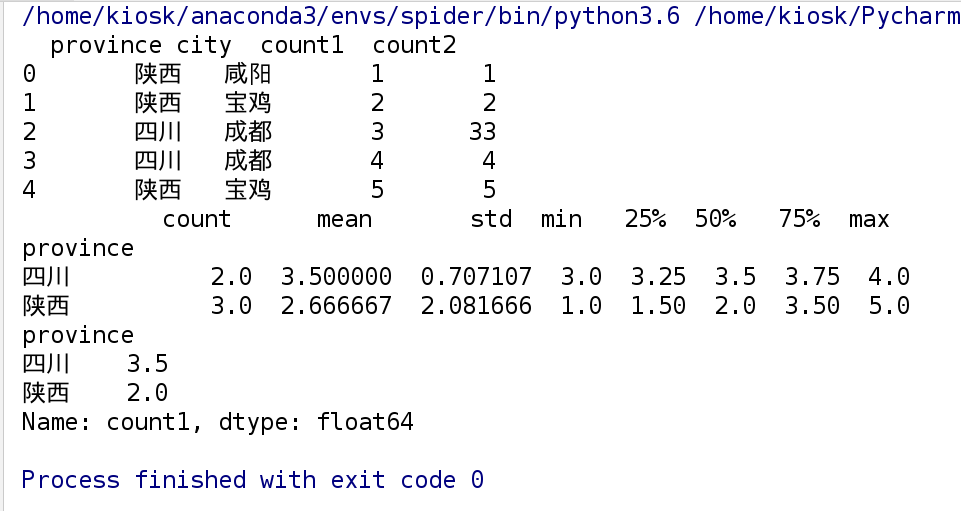import pandas as pd

df = pd.DataFrame(
{'province': ['陕西', '陕西', '四川', '四川', '陕西'],
'city': ['咸阳', '宝鸡', '成都', '成都', '宝鸡'],
'count1': [1, 2, 3, 4, 5],
'count2': [1, 2, 33, 4, 5]
}
)
print(df)
# 根据城市统计分析cpunt1的信息;
grouped = df['count1'].groupby(df['city'])
print(grouped.max())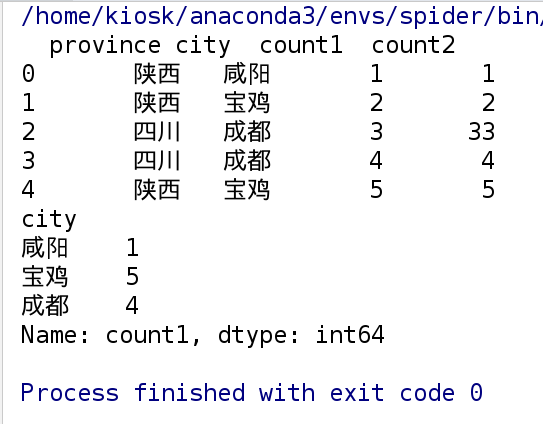import pandas as pd

df = pd.DataFrame(
{'province': ['陕西', '陕西', '四川', '四川', '陕西'],
'city': ['咸阳', '宝鸡', '成都', '成都', '宝鸡'],
'count1': [1, 2, 3, 4, 5],
'count2': [1, 2, 33, 4, 5]
}
)
print(df)
# 指定多个key值进行分类聚合;
grouped = df['count1'].groupby([df['province'], df['city']])
print(grouped)
print(grouped.max())
# 统计各个城市的count1的总和
print(grouped.sum())
# 统计各个城市出现的次数
print(grouped.count())
#  通过unstack方法， 实现层次化的索引;
# 将province这个大分组当作行索引，city这个小分组当作列索引
print(grouped.max().unstack())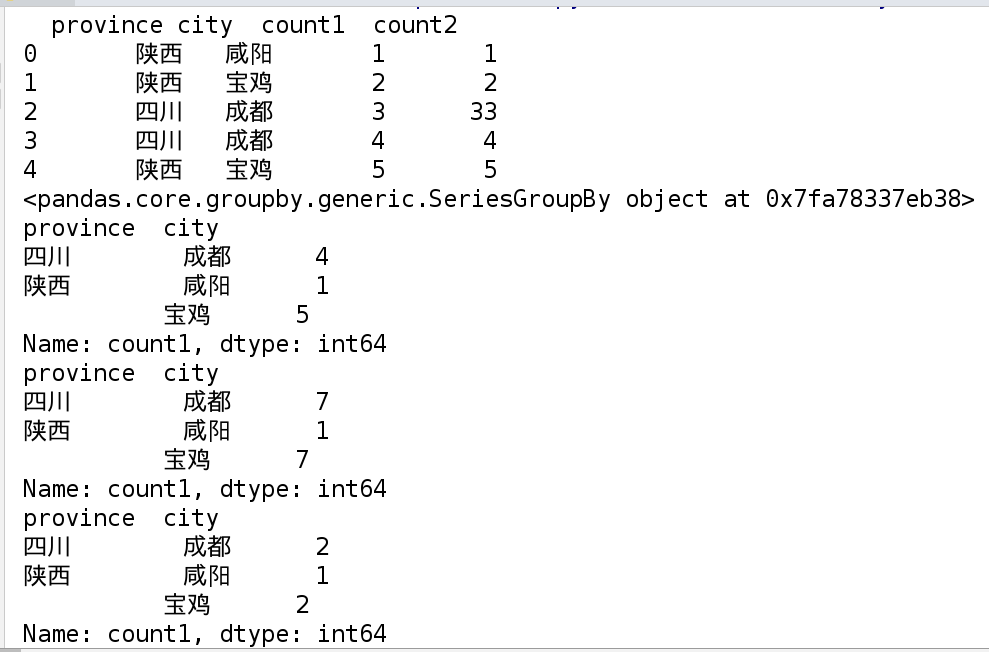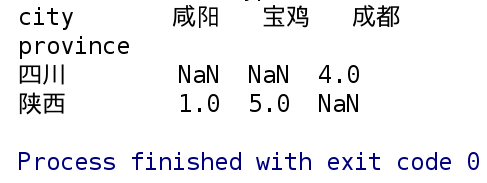案例1_商品数据分析

文件名称： doc/chipo.csv
文件描述: 每列数据分别代表如下: 订单编号, 订单数量, 商品名称， 商品详细选择项， 商品单价

需求1：

从文件中读取所有的数据;
获取数据中所有的商品名称；
跟据商品的价格进行排序， 降序，将价格最高的20件产品信息写入mosthighPrice.xlsx文件中;

import pandas as pd
import numpy as np
"""
需求1：
1). 从文件中读取所有的数据;
2). 获取数据中所有的商品名称；
"""
# 读取文件信息
# 去掉索引列
df.pop('Unnamed: 0')
# 取出所有商品名称
itemName=df['item_name'].values
print(itemName)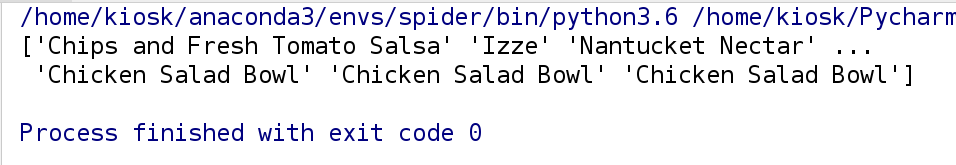# 3).跟据商品的价格进行排序， 降序，将价格最高的20件产品信息写入mosthighPrice.xlsx文件中;
# 取出商品单价，并去掉$符号，再转成浮点型 price=df['item_price'].str.strip('$').astype(np.float)
# 添加一列处理好的单价信息
df['price']=price
# 将商品按添加的一列单价信息进行降序排序，并取前20行
# 去掉添加的一列单价信息
df.pop('price')
df.to_excel('/tmp/item.xlsx')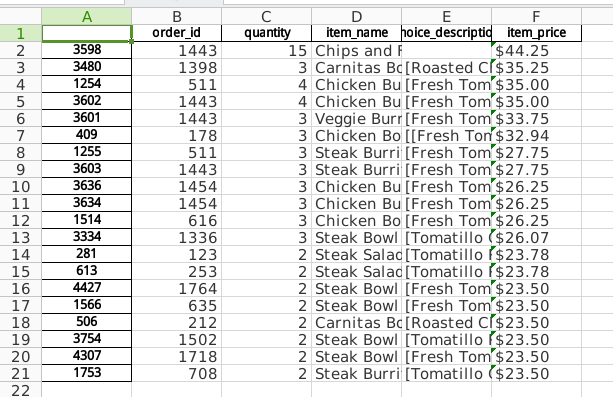需求2：

统计列[item_name]中每种商品出现的频率，绘制柱状图
(购买次数最多的商品排名-绘制前5条记录)
根据列 [odrder_id] 分组，求出每个订单花费的总金额。
根据每笔订单的总金额和其商品的总数量画出散点图。

# 1). 统计列[item_name]中每种商品出现的频率，绘制柱状图(购买次数最多的商品排名-绘制前5条记录)
import pandas as pd
from pyecharts import Bar
df.pop('Unnamed: 0')
# 将数据按商品名称分组，并求每组商品数量的总和
count=df['quantity'].groupby(by=df['item_name']).sum()
# 将数据降序排序，并取前5行
bar=Bar('柱状图')
bar.render('bar.html')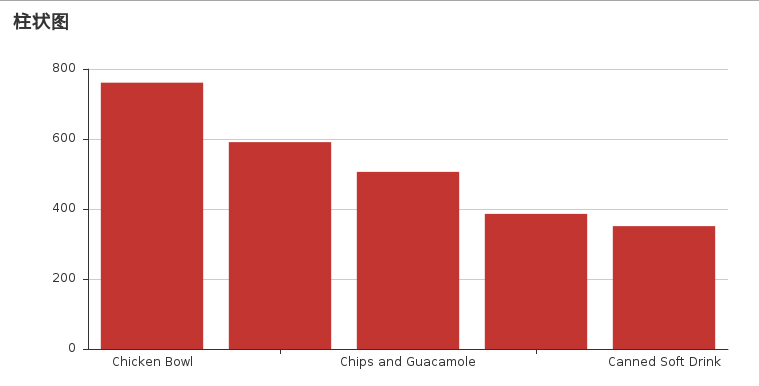# 2). 根据列 [odrder_id] 分组，求出每个订单花费的总金额。
import pandas as pd
import numpy as np
df.pop('Unnamed: 0')
# 取出商品单价，并去掉$符号，再转成浮点型 price=df['item_price'].str.strip('$').astype(np.float)
# 添加一列处理好的单价信息
df['price']=price
# 将数据的quantity列和price列相乘得到每一行总价
all_price=df['quantity']*df['price']
# 将总价信息添加到数据的一列中
df['all_price']=all_price
# 按订单号进行分组，求每个订单号的总价
data=df['all_price'].groupby(by=df['order_id']).sum()
print(data)
# 3). 根据每笔订单的总金额和其商品的总数量画出散点图。
# 按订单号进行分组，求每个订单号的商品总数
count=df['quantity'].groupby(by=df['order_id']).sum()
sc=Scatter('散点图')
sc.render('scatter.html')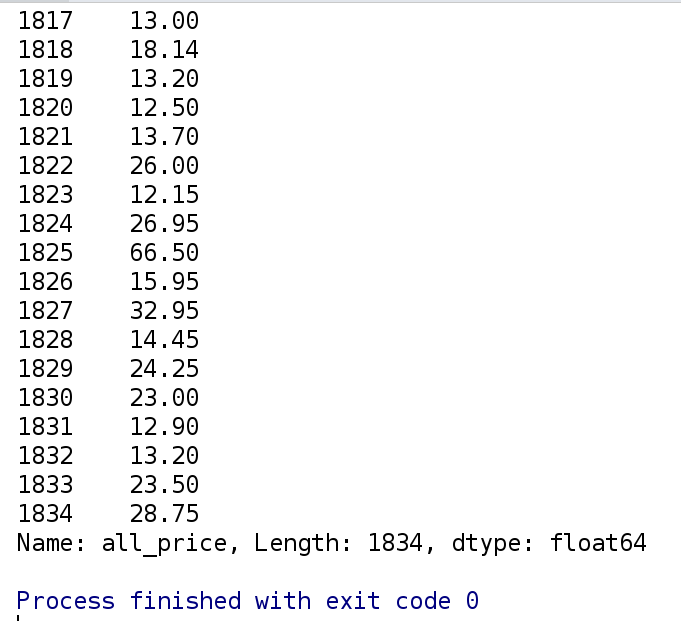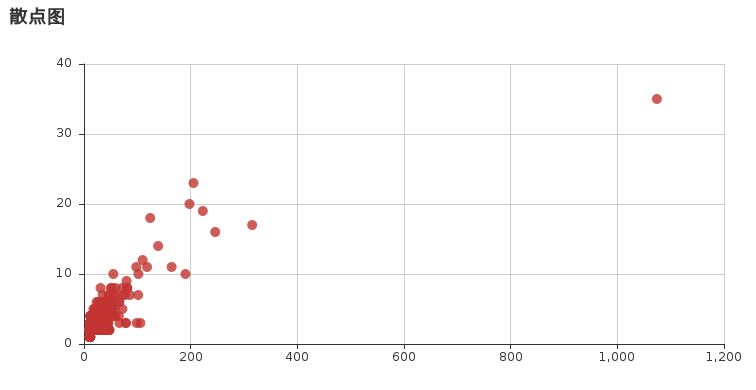字符串的操作
在一维数组Series中有.str方法，可以对数组内的元素进行字符串操作
import pandas as pd
import numpy as np

series1= pd.Series(['$A:1', '$B:2', '$C:3', np.nan, '$cat:3'])
print(series1)

# 将所有的字母转换为小写字母， 除了缺失值
print(series1.str.lower())

# 将所有的字母转换为大写字母， 除了缺失值
print(series1.str.upper())

# 分离
print(series1.str.split(":"))

# 去掉左右两端的某个字符
print(series1.str.strip('\$'))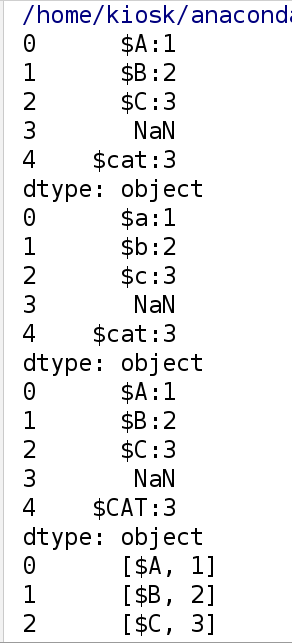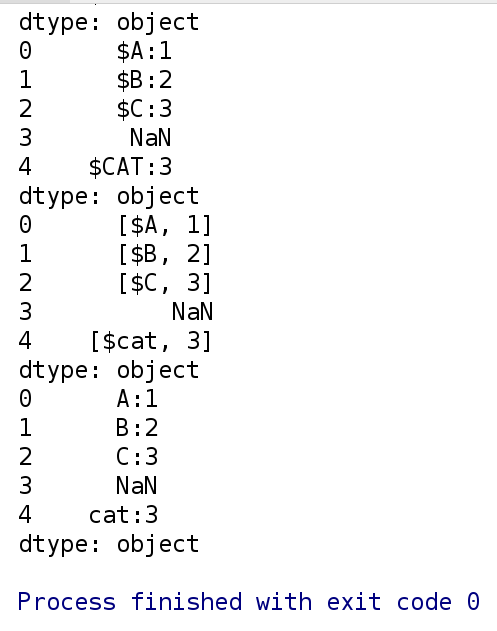案例2_消费金额和小费之间的关联与性别和吸烟与否的关系

文件名称: doc/tips.csv
文件内容: 总消费金额， 小费金额， 性别， 是否抽烟， 日期， 时间， 星期

需求:

分别吸烟顾客与不吸烟顾客的消费金额与小费之间的散点图;
女性与男性中吸烟与不吸烟顾客的消费金额与小费之间的散点图关系;

# 分别吸烟顾客与不吸烟顾客的消费金额与小费之间的散点图;
import numpy as np
from matplotlib import pyplot as plt
import pandas as pd
from pyecharts import EffectScatter
smoker=df[df.smoker=='Yes']
noSmoker=df[df.smoker=='No']
smoker_y=smoker.total_bill
smoker_x=smoker.tip
noSmoker_x=noSmoker.tip
noSmoker_y=noSmoker.total_bill
es=EffectScatter('消费金额于小费之间的关系')
es.render()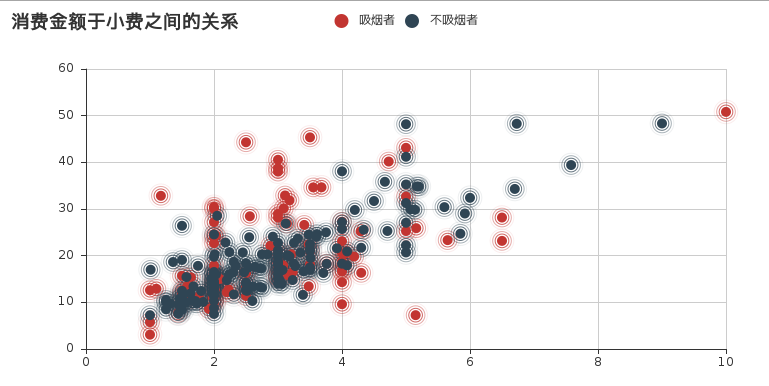# 女性与男性中吸烟与不吸烟顾客的消费金额与小费之间的散点图关系;
import numpy as np
from matplotlib import pyplot as plt
import pandas as pd
from pyecharts import EffectScatter
male=df[df.sex=='Male']
female=df[df.sex=='Female']
male_smoker=male[male.smoker=='Yes']
male_noSmoker=male[male.smoker=='No']
male_smoker_x=male_smoker.tip
male_smoker_y=male_smoker.total_bill
male_noSmoker_x=male_noSmoker.tip
male_noSmoker_y=male_noSmoker.total_bill
female_smoker=female[female.smoker=='Yes']
female_noSmoker=female[female.smoker=='No']
female_smoker_x=female_smoker.tip
female_smoker_y=female_smoker.total_bill
female_noSmoker_x=female_noSmoker.tip
female_noSmoker_y=female_noSmoker.total_bill
es=EffectScatter('男女吸烟与不吸烟的消费金额和小费关系图')
es.render()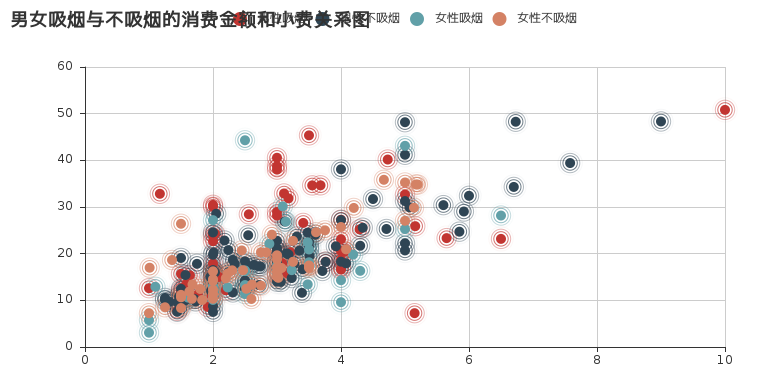展开全文• 1.BP神经网络简介 BP神经网络是1986年由Rumelhart和McClelland为首的科学家提出的概念，是一种按照逆向传播算法训练的多层前馈神经网络，是目前应用最广泛的神经网络。 优点：具有任意复杂的模式分类能力和优良的...
0.目录

1.BP神经网络简介

2.前期理论准备

2.算法数学原理

（一）符号说明

（二）公式推导

3.python实现（python3编程实现）

（一）sigmoid函数

（二）BP主函数实现

4.数据格式

1.BP神经网络简介

BP神经网络是1986年由Rumelhart和McClelland为首的科学家提出的概念，是一种按照逆向传播算法训练的多层前馈神经网络，是目前应用最广泛的神经网络。

目录

0.目录

1.BP神经网络简介

2.前期理论准备

2.算法数学原理

（一）符号说明

（二）公式推导

3.python实现（python3编程实现）

（一）sigmoid函数

（二）BP主函数实现

4.数据格式

优点：

1.具有任意复杂的模式分类能力和优良的多维函数映射能力，解决了简单感知器不能解决的异或问题的问题（参考博客：https://www.jianshu.com/p/a25788130897 或 https://www.cnblogs.com/xym4869/p/11282469.html）
2.从结构上讲，BP神经网络具有输入层、隐含层和输出层
3.从本质上讲，BP算法就是以网络误差平方目标函数、采用梯度下降法来计算目标函数的最小值。基本BP算法包括信号的前向传播和误差的反向传播两个过程。
缺点：

1.学习速度慢，即使是一个简单的过程，也需要几百次甚至上千次的学习才能收敛。
2.容易陷入局部极小值
3.网络层数、神经元个数的选择没有相应的理论指导
4.网络推广能力有限。
应用：

1.函数逼近
2.模式识别
3.分类
4.数据压缩

2.前期理论准备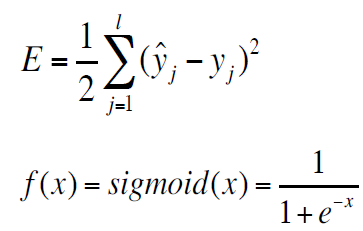网络训练的目标：找到合适的权值和阈值，使得误差E最小。

sigmoid函数：在信息科学当中，由于其单增以及其反函数单增等性质，sigmoid函数常被用作神经网络的阈值函数，将变量映射当0和1之间。（该函数的对x的求导也应该理解）

2.算法数学原理

（一）符号说明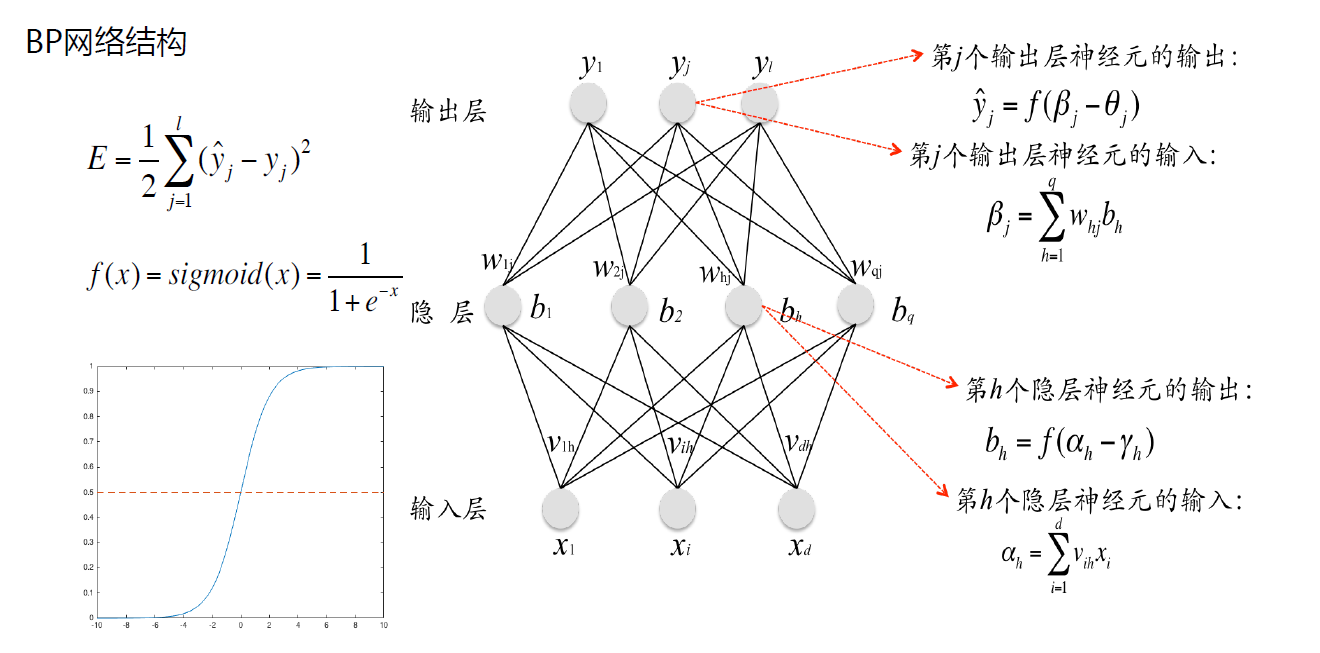Xi: 输入信号。

Xd: 隐层的阈值（是从求和函数中-θ中分离出的-1）。

Vih: 第h个隐层神经元所对应输入信号Xi的权值。

αh: 第h个隐层神经元的输入。

-γh=--1*γh：隐层神经元的阈值。

bh: 第h个隐层神经元的输入。

ωhj: 第j个输出层神经元所对应的隐层神经元输出bh的权值。

-θj=-1*θj:  输出层神经元的阈值（bq）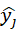：第j个输出层神经元的输出（预测输出值，yj为真实值）

（二）公式推导

通过公式变换可得输出层权值与阈值的变化量：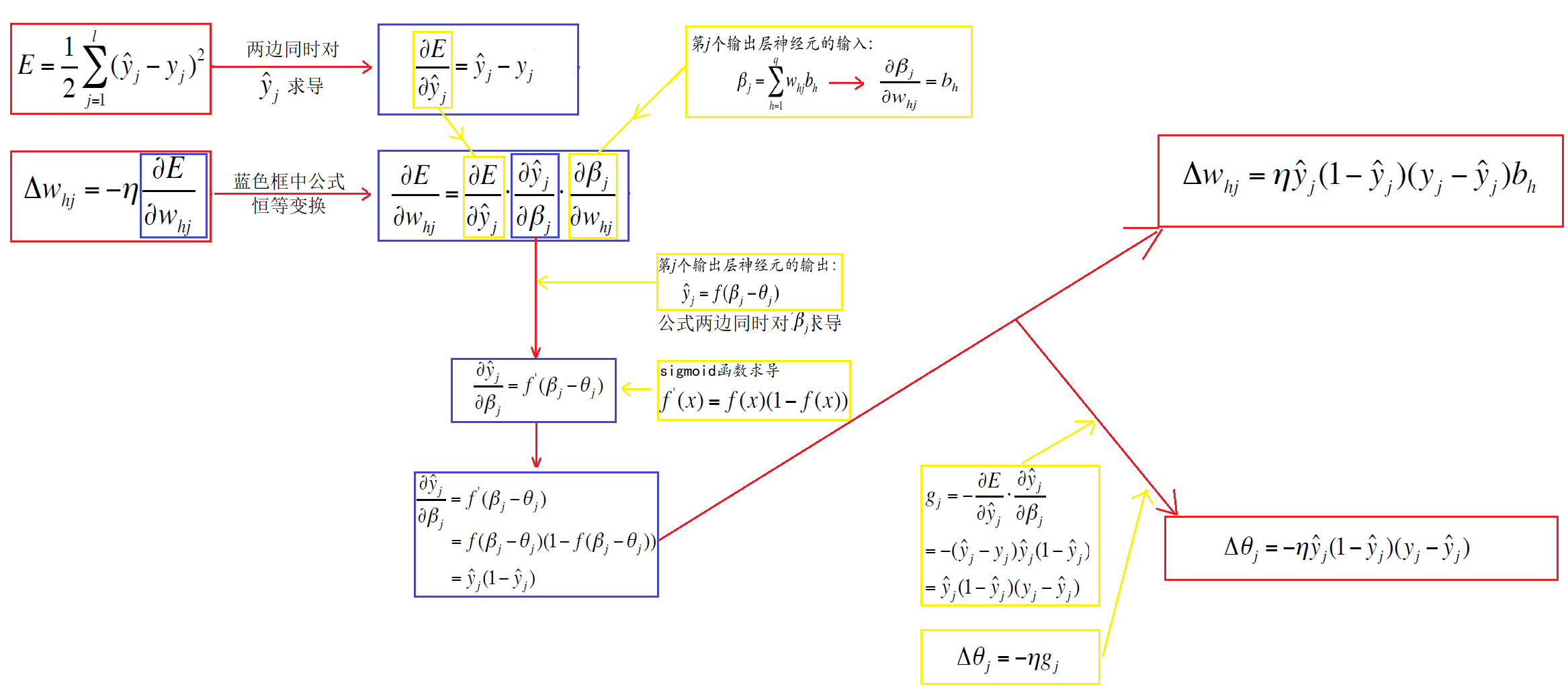同理可得隐层权值和阈值的变化量：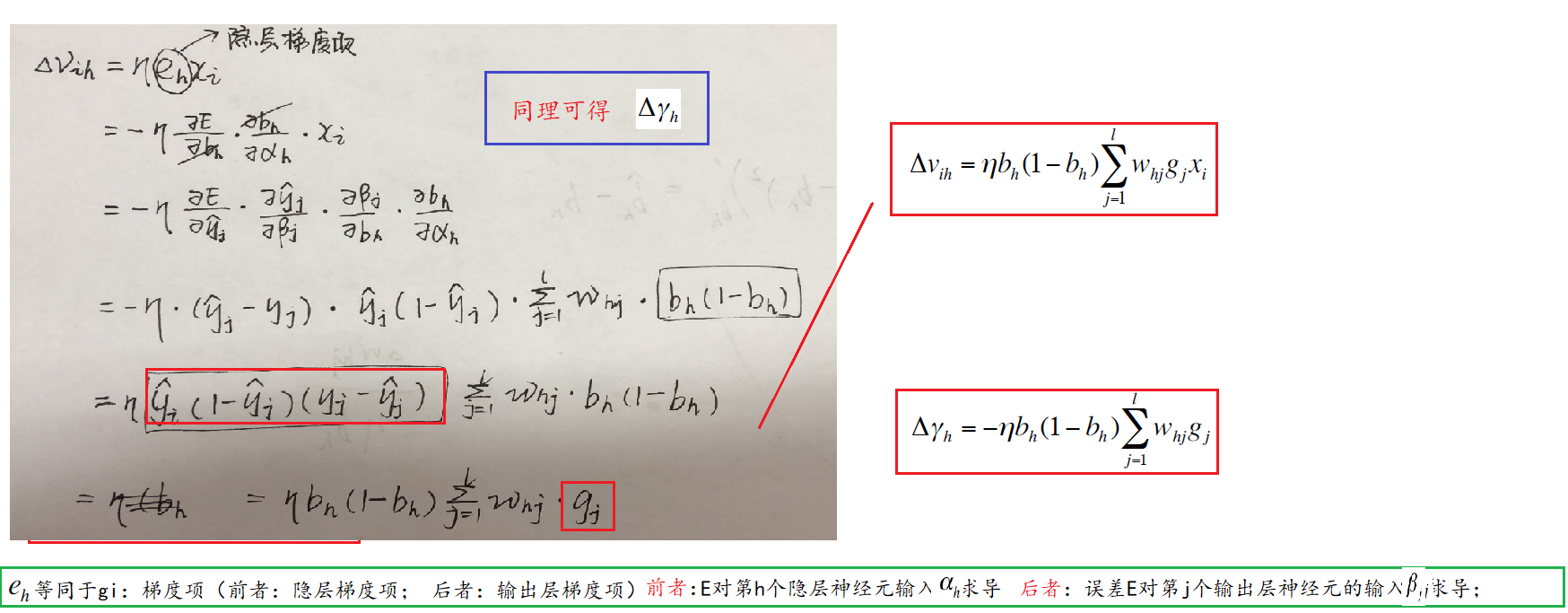3.python实现（python3编程实现）

（一）sigmoid函数

def sigmoid(x):
"""
隐含层和输出层对应的函数法则
"""
return 1/(1+np.exp(-x))


（二）BP主函数实现

def BP(data_tr, data_te, maxiter=600):

# --pandas是基于numpy设计的，效率略低
# 为提高处理效率，转换为数组
data_tr, data_te = np.array(data_tr), np.array(data_te)

# --隐层输入
# -1： 代表的是隐层的阈值
net_in = np.array([0.0, 0, -1])
w_mid = np.random.rand(3, 4)          # 隐层权值阈值（-1x其中一个值：阈值）

# 输出层输入
# -1：代表输出层阈值
out_in = np.array([0.0, 0, 0, 0, -1])
w_out = np.random.rand(5)             # 输出层权值阈值（-1x其中一个值：阈值）
delta_w_out = np.zeros()           # 存放输出层权值阈值的逆向计算误差
delta_w_mid = np.zeros([3, 4])        # 存放因此能权值阈值的逆向计算误差
yita = 1.75                           # η： 学习速率
Err = np.zeros([maxiter])             # 记录总体样本每迭代一次的错误率

# 1.样本总体训练的次数
for it in range(maxiter):

# 衡量每一个样本的误差
err = np.zeros([len(data_tr)])

# 2.训练集训练一遍
for j in range(len(data_tr)):
net_in[:2] = data_tr[j, :2]                       # 存储当前对象前两个属性值
real = data_tr[j, 2]

# 3.当前对象进行训练
for i in range(4):
out_in[i] = sigmoid(sum(net_in*w_mid[:, i]))  # 计算输出层输入
res = sigmoid(sum(out_in * w_out))                # 获得训练结果

err[j] = abs(real - res)

# --先调节输出层的权值与阈值
delta_w_out = yita*res*(1-res)*(real-res)*out_in  # 权值调整
delta_w_out = -yita*res*(1-res)*(real-res)     # 阈值调整
w_out = w_out + delta_w_out

# --隐层权值和阈值的调节
for i in range(4):
# 权值调整
delta_w_mid[:, i] = yita * out_in[i] * (1 - out_in[i]) * w_out[i] * res * (1 - res) * (real - res) * net_in
# 阈值调整
delta_w_mid[2, i] = -yita * out_in[i] * (1 - out_in[i]) * w_out[i] * res * (1 - res) * (real - res)
w_mid = w_mid + delta_w_mid
Err[it] = err.mean()
plt.plot(Err)
plt.show()

# 存储预测误差
err_te = np.zeros()

# 预测样本100个
for j in range(100):
net_in[:2] = data_te[j, :2]                         # 存储数据
real = data_te[j, 2]                                # 真实结果

# net_in和w_mid的相乘过程
for i in range(4):
# 输入层到隐层的传输过程
out_in[i] = sigmoid(sum(net_in*w_mid[:, i]))
res = sigmoid(sum(out_in*w_out))                    # 网络预测结果输出
err_te[j] = abs(real-res)                           # 预测误差
print('res:', res, ' real:', real)

plt.plot(err_te)
plt.show()

if "__main__" == __name__:

# 1.读取样本
BP(data_tr, data_te, maxiter=600)

4.数据格式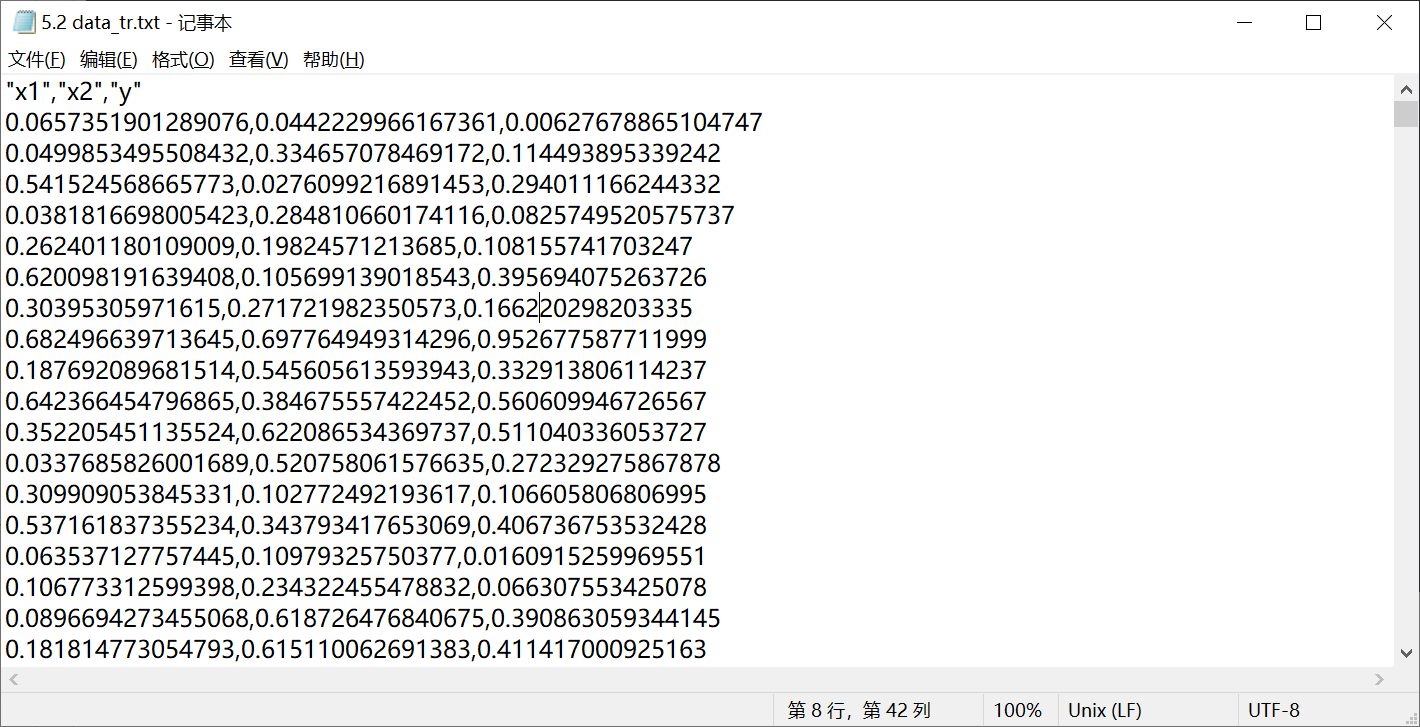展开全文BP神经网络 神经网络公式推导 神经网络
• Pandas 的名称来自于面板数据（panel data）和python数据分析 （data analysis） 。panel data是经济学中关于多维数据集的一个术语，在Pandas中也提供了panel的数据类型。 在我看来，对于 Numpy 以及 Matplotlib ...
分享一个朋友的人工智能教程。零基础！通俗易懂！风趣幽默！还带黄段子！大家可以看看是否对自己有帮助：点击打开
docker/kubernetes入门视频教程

起步
Pandas最初被作为金融数据分析工具而开发出来，因此 pandas 为时间序列分析提供了很好的支持。 Pandas 的名称来自于面板数据（panel data）和python数据分析 （data analysis） 。panel data是经济学中关于多维数据集的一个术语，在Pandas中也提供了panel的数据类型。
在我看来，对于 Numpy 以及 Matplotlib ，Pandas可以帮助创建一个非常牢固的用于数据挖掘与分析的基础。而Scipy当然是另一个主要的也十分出色的科学计算库。本文使用python3.6来编写代码。
安装与导入
通过pip进行安装: pip install pandas
导入：
import pandas as pd

Pandas的数据类型
Pandas基于两种数据类型： series 与 dataframe 。
Series
一个series是一个一维的数据类型，其中每一个元素都有一个标签。类似于Numpy中元素带标签的数组。其中，标签可以是数字或者字符串。
import numpy as np
import pandas as pd

s = pd.Series([1, 2, 5, np.nan, 6, 8])
print(s)

输出：
0    1.0
1    2.0
2    5.0
3    NaN
4    6.0
5    8.0
dtype: float64

DataFrame
一个dataframe是一个二维的表结构。Pandas的dataframe可以存储许多种不同的数据类型，并且每一个坐标轴都有自己的标签。你可以把它想象成一个series的字典项。
创建一个 DateFrame：
#创建日期索引序列
dates =pd.date_range('20130101', periods=6)
print(type(dates))
#创建Dataframe，其中 index 决定索引序列，columns 决定列名
df =pd.DataFrame(np.random.randn(6,4), index=dates, columns=list('ABCD'))
print(df)

输出：
<class 'pandas.core.indexes.datetimes.DatetimeIndex'>
A         B         C         D
2013-01-01  0.406575 -1.356139  0.188997 -1.308049
2013-01-02 -0.412154  0.123879  0.907458  0.201024
2013-01-03  0.576566 -1.875753  1.967512 -1.044405
2013-01-04  1.116106 -0.796381  0.432589  0.764339
2013-01-05 -1.851676  0.378964 -0.282481  0.296629
2013-01-06 -1.051984  0.960433 -1.313190 -0.093666

字典创建 DataFrame
df2 =pd.DataFrame({'A' : 1.,
'B': pd.Timestamp('20130102'),
'C': pd.Series(1,index=list(range(4)),dtype='float32'),
'D': np.array(*4,dtype='int32'),
'E': pd.Categorical(["test","train","test","train"]),
'F':'foo' })

print(df2)

输出：
     A          B    C  D      E    F
0  1.0 2013-01-02  1.0  3   test  foo
1  1.0 2013-01-02  1.0  3  train  foo
2  1.0 2013-01-02  1.0  3   test  foo
3  1.0 2013-01-02  1.0  3  train  foo

将文件数据导入Pandas
df =pd.read_csv("Average_Daily_Traffic_Counts.csv", header=0)

数据源可以是 英国政府数据 或 美国政府数据 来获取数据源。当然， Kaggle 是另一个好用的数据源。
选择/切片
df[‘column_name’] ，df[row_start_index, row_end_index] 选取指定整列数据
df['name']   # 选取一列，成一个series
df[['name']]  # 选取一列，成为一个dataframe
df[['name','gender']] #选取多列，多列名字要放在list里
df[0:]  #第0行及之后的行，相当于df的全部数据，注意冒号是必须的
df[:2]  #第2行之前的数据（不含第2行）
df[0:1] #第0行
df[1:3] #第1行到第2行（不含第3行）
df[-1:] #最后一行
df[-3:-1] #倒数第3行到倒数第1行（不包含最后1行即倒数第1行，这里有点烦躁，因为从前数时从第0行开始，从后数就是-1行开始，毕竟没有-0）

loc，在知道列名字的情况下，df.loc[index,column] 选取指定行，列的数据
# df.loc[index, column_name],选取指定行和列的数据
df.loc[0,'name'] # 'Snow'
df.loc[0:2, ['name','age']]          #选取第0行到第2行，name列和age列的数据, 注意这里的行选取是包含下标的。
df.loc[[2,3],['name','age']]         #选取指定的第2行和第3行，name和age列的数据
df.loc[df['gender']=='M','name']     #选取gender列是M，name列的数据
df.loc[df['gender']=='M',['name','age']] #选取gender列是M，name和age列的数据

iloc，在column name特别长或者index是时间序列等各种不方便输入的情况下，可以用iloc (i = index), iloc完全用数字来定位 iloc[row_index, column_index]
df.iloc[0,0]        #第0行第0列的数据，'Snow'
df.iloc[1,2]        #第1行第2列的数据，32
df.iloc[[1,3],0:2]  #第1行和第3行，从第0列到第2列（不包含第2列）的数据
df.iloc[1:3,[1,2]   #第1行到第3行（不包含第3行），第1列和第2列的数据

索引汇总
pandas的索引函数主要有三种：
loc 标签索引，行和列的名称
iloc 整型索引（绝对位置索引），绝对意义上的几行几列，起始索引为0
ix 是 iloc 和 loc的合体
at是loc的快捷方式
iat是iloc的快捷方式
# 选择单独的一列，返回 Serires，与 df.A 效果相当。
df['A']

# 位置切片
df[0:3]

# 索引切片
df['20130102':'20130104']

#　通过标签选择
df.loc[dates]

# 对多个轴同时通过标签进行选择
df.loc[:,['A','B']]

# 获得某一个单元的数据
df.loc[dates,'A']
# 或者
df.at[dates,'A']#　速度更快的做法

# 通过位置进行选择
df.iloc

# 切片
df.iloc[3:5,0:2]

# 列表选择
df.iloc[[1,2,4],[0,2]]

# 获得某一个单元的数据
df.iloc[1,1]
# 或者
df.iat[1,1]# 更快的做法

# 布尔索引
df[df.A > 0]

# 获得大于零的项的数值
df[df > 0]

# isin 过滤
df2[df2['E'].isin(['two','four'])]

赋值
# 新增一列，根据索引排列
s1 =pd.Series([1,2,3,4,5,6], index=pd.date_range('20130102', periods=6))
df['F']=s1

# 缺省项
# 在 pandas 中使用 np.nan 作为缺省项的值。
df1 =df.reindex(index=dates[0:4], columns=list(df.columns)+['E'])
df1.loc[dates:dates,'E']=1

# 删除所有带有缺省项的行
df1.dropna(how='any')

# 填充缺省项
df1.fillna(value=5)

# 获得缺省项的布尔掩码
pd.isnull(df1)

算数运算
两个dataframe 矩阵相加、相减、相乘、相除，会对两个矩阵行索引（包括行索引名称和行索引值）和列索引相同的两个对应元素做运算。
观察操作
# 观察开头的数据

# 观察末尾的数据
df.tail(3)

# 显示索引
df.index

# 显示列
df.columns

# 显示底层 numpy 结构
df.values

# DataFrame 的基本统计学属性预览
df.describe()
"""
A  B  C  D
count 6.000000 6.000000 6.000000 6.000000 #数量
mean 0.073711 -0.431125 -0.687758 -0.233103 #平均值
std 0.843157 0.922818 0.779887 0.973118 #标准差
min -0.861849 -2.104569 -1.509059 -1.135632 #最小值
25% -0.611510 -0.600794 -1.368714 -1.076610 #正态分布 25%
50% 0.022070 -0.228039 -0.767252 -0.386188 #正态分布 50%
75% 0.658444 0.041933 -0.034326 0.461706 #正态分布 75%
max 1.212112 0.567020 0.276232 1.071804 #最大值
"""

# 转置
df.T

# 根据某一轴的索引进行排序
df.sort_index(axis=1, ascending=False)

# 根据某一列的数值进行排序
df.sort(columns='B')

统计
count                      非NA值的数量
describe                  针对Series或各DataFrame列计算汇总统计
min,max                 计算最小值和最大值
argmin,argmax        计算能够获取到最小值和最大值的索引位置（整数)
idxmin,idxmax         计算能够获取到最小值和最大值的索引值
quantile                   计算样本的分位数（0到 1）
sum                        值的总和
mean                      值的平均数， a.mean() 默认对每一列的数据求平均值；若加上参数a.mean(1)则对每一行求平均值
media                      值的算术中位数（50%分位数)
var                          样本值的方差
std                        样本值的标准差
skew                     样本值的偏度（三阶矩）
kurt                       样本值的峰度（四阶矩）
cumsum                 样本值的累计和
cummin,cummax    样本值的累计最大值和累计最小
cumprod                样本值的累计积
diff                        计算一阶差分（对时间序列很有用)
pct_change            计算百分数变化

# 求平均值
df.mean()
"""
A -0.004474
B -0.383981
C -0.687758
D 5.000000
F 3.000000
dtype: float64
"""

# 指定轴上的平均值
df.mean(1)

# 不同维度的 pandas 对象也可以做运算，它会自动进行对应，shift 用来做对齐操作。
s = pd.Series([1,3,5,np.nan,6,8], index=dates).shift(2)
"""
2013-01-01NaN
2013-01-02NaN
2013-01-031
2013-01-043
2013-01-055
2013-01-06NaN
Freq: D, dtype: float64
"""

# 对不同维度的 pandas 对象进行减法操作
df.sub(s, axis='index')
"""
A B C D F
2013-01-01NaN NaN NaN NaN NaN
2013-01-02NaN NaN NaN NaN NaN
2013-01-03-1.861849-3.104569-1.49492941
2013-01-04-2.278445-3.706771-4.03957520
2013-01-05-5.424972-4.432980-4.7237680-1
2013-01-06NaN NaN NaN NaN NaN
"""

函数应用
# 累加
df.apply(np.cumsum)

直方图
s =pd.Series(np.random.randint(0,7, size=10))
s.value_counts()
"""
4 5
6 2
2 2
1 1
dtype: int64
String Methods
"""

字符处理
s =pd.Series(['A','B','C','Aaba','Baca', np.nan,'CABA','dog','cat'])
s.str.lower()
"""
0 a
1 b
2 c
3 aaba
4 baca
5 NaN
6 caba
7 dog
8 cat
dtype: object
"""

合并
使用 concat() 连接 pandas 对象:
df =pd.DataFrame(np.random.randn(10,4))
"""
0  1  2  3
0 -0.548702 1.467327 -1.015962 -0.483075
1 1.637550 -1.217659 -0.291519 -1.745505
2 -0.263952 0.991460 -0.919069 0.266046
3 -0.709661 1.669052 1.037882 -1.705775
4 -0.919854 -0.042379 1.247642 -0.009920
5 0.290213 0.495767 0.362949 1.548106
6 -1.131345 -0.089329 0.337863 -0.945867
7 -0.932132 1.956030 0.017587 -0.016692
8 -0.575247 0.254161 -1.143704 0.215897
9 1.193555 -0.077118 -0.408530 -0.862495
"""

pieces =[df[:3], df[3:7], df[7:]]
pd.concat(pieces)
"""
0  1  2  3
0 -0.548702 1.467327 -1.015962 -0.483075
1 1.637550 -1.217659 -0.291519 -1.745505
2 -0.263952 0.991460 -0.919069 0.266046
3 -0.709661 1.669052 1.037882 -1.705775
4 -0.919854 -0.042379 1.247642 -0.009920
5 0.290213 0.495767 0.362949 1.548106
6 -1.131345 -0.089329 0.337863 -0.945867
7 -0.932132 1.956030 0.017587 -0.016692
8 -0.575247 0.254161 -1.143704 0.215897
9 1.193555 -0.077118 -0.408530 -0.862495
"""

join 合并：
left =pd.DataFrame({'key': ['foo','foo'],'lval': [1,2]})
right =pd.DataFrame({'key': ['foo','foo'],'rval': [4,5]})
pd.merge(left, right, on='key')
"""
key lval rval
0 foo 1 4
1 foo 1 5
2 foo 2 4
3 foo 2 5
"""

追加
在 dataframe 数据后追加行
df =pd.DataFrame(np.random.randn(8,4), columns=['A','B','C','D'])
s =df.iloc
df.append(s, ignore_index=True)

分组
分组常常意味着可能包含以下的几种的操作中一个或多个
依据一些标准分离数据
对组单独地应用函数
将结果合并到一个数据结构中
df =pd.DataFrame({'A': ['foo','bar','foo','bar','foo','bar','foo','foo'],
'B': ['one','one','two','three','two','two','one','three'],
'C': np.random.randn(8),
'D': np.random.randn(8)})
print('源数据集：\n',df)

df1 = df.groupby('A').sum()
print('A分组：\n',df1)

df2 = df.groupby(['A','B']).sum()
print('AB分组：\n',df2)

输出结果
源数据集：
A      B         C         D
0  foo    one  0.423062 -0.813870
1  bar    one -1.058636 -0.943536
2  foo    two -0.843569 -0.611338
3  bar  three  0.933234 -1.425916
4  foo    two -1.145840  0.643593
5  bar    two  1.057359 -1.049414
6  foo    one -0.387183  1.056451
7  foo  three  1.923139 -1.184541
A分组：
C         D
A
bar  0.931957 -3.418865
foo -0.030391 -0.909705
AB分组：
C         D
A   B
bar one   -1.058636 -0.943536
three  0.933234 -1.425916
two    1.057359 -1.049414
foo one    0.035879  0.242581
three  1.923139 -1.184541
two   -1.989409  0.032255

分组时，组内运算
代表运算的字符串包括‘sum’、‘mean’、‘min’、‘max’、‘count’
pd3 = pd3.groupby('a').agg('sum').reset_index()

或者自定义函数
# # 或自定义函数不需要参数，则x是serise，如果x有自定参数，则x为DataFrame
def funname(x,name):
print(name)
print(type(x),'\n',x)
return 2

pd3 = pd3.groupby('a').agg(funname,'aaa').reset_index()

数据透视表
df =pd.DataFrame({'A': ['one','one','two','three']*3,
'B': ['A','B','C']*4,
'C': ['foo','foo','foo','bar','bar','bar']*2,
'D': np.random.randn(12),
'E': np.random.randn(12)})

# 生成数据透视表
pd.pivot_table(df, values='D', index=['A','B'], columns=['C'])
"""
C  bar foo
A B
one A -0.773723 1.418757
B -0.029716 -1.879024
C -1.146178 0.314665
three A 1.006160 NaN
B NaN -1.035018
C 0.648740 NaN
two A NaN 0.100900
B -1.170653 NaN
C NaN 0.536826
"""

时间序列
pandas 拥有既简单又强大的频率变换重新采样功能，下面的例子从 1次/秒 转换到了 1次/5分钟：
rng =pd.date_range('1/1/2012', periods=100, freq='S')
ts =pd.Series(np.random.randint(0,500,len(rng)), index=rng)
ts.resample('5Min', how='sum')
"""
2012-01-01 25083
Freq: 5T, dtype: int32
"""

# 本地化时区表示
rng =pd.date_range('3/6/2012 00:00', periods=5, freq='D')
ts =pd.Series(np.random.randn(len(rng)), rng)
"""
2012-03-06 0.464000
2012-03-07 0.227371
2012-03-08 -0.496922
2012-03-09 0.306389
2012-03-10 -2.290613
Freq: D, dtype: float64
"""

ts_utc =ts.tz_localize('UTC')
"""
2012-03-06 00:00:00+00:00 0.464000
2012-03-07 00:00:00+00:00 0.227371
2012-03-08 00:00:00+00:00 -0.496922
2012-03-09 00:00:00+00:00 0.306389
2012-03-10 00:00:00+00:00 -2.290613
Freq: D, dtype: float64
"""

# 转换为周期
ps =ts.to_period()

# 转换为时间戳
ps.to_timestamp()

分类
df =pd.DataFrame({"id":[1,2,3,4,5,6],"raw_grade":['a','b','b','a','a','e']})

# 将 raw_grades 转换成 Categoricals 类型
"""
0 a
1 b
2 b
3 a
4 a
5 e
Categories (3, object): [a, b, e]
"""

# 重命名分类

# 根据分类的顺序对数据进行排序
"""
1 2   b  good
2 3   b  good
0 1   a very good
3 4   a very good
4 5   a very good
"""

作图
ts =pd.Series(np.random.randn(1000), index=pd.date_range('1/1/2000', periods=1000))
ts =ts.cumsum()
ts.plot()

数据IO
# 从 csv 文件读取数据

# 保存到 csv 文件
df.to_csv('foo.csv')

# 读取 excel 文件

# 保存到 excel 文件
df.to_excel('foo.xlsx', sheet_name='Sheet1')

python | pandas | 移动窗口函数rolling
https://blog.csdn.net/xxzhangx/article/details/76938053
总结
以上就是这篇文章的全部内容了，希望本文的内容对大家学习或者使用python能带来一定的帮助，如果有疑问大家可以留言交流。


展开全文数据分析 大数据 数据挖掘
• 1.拆分列 将原数据的指定列按照列的内容拆分为新的列（相当于Excel中的数据透视表） 有原始数据格式如下： 需要将type列拆分为按照type值为列，值为monetary值的表，如下： 代码可以写为： #将原数据的指定列按照...
1.拆分列

将原数据的指定列按照列的内容拆分为新的列（相当于Excel中的数据透视表）

有原始数据格式如下：需要将type列拆分为按照type值为列，值为monetary值的表，如下：代码可以写为：
#将原数据的指定列按照列的内容拆分为新的列
def pivot():
table=pd.DataFrame({'cust_id':[10001,10001,10002,10002,10003],
'type':['Normal','Special_offer','Normal','Special_offer','Special_offer'],
'Monetary':[3608,420,1894,3503,4567]})
print(table)
print('-'*60)
table_pivot=table.pivot_table(values='Monetary',index='cust_id',columns='type',fill_value=0,aggfunc='sum')
print(table_pivot)

2.堆叠列

是拆分列的反操作，当存在表示列中有多个数值变量的时候，可以通过堆叠列将多列的数据堆积在一起

有原始数据格式如下：需要将normal和special_offer合并为type列，值为各自值的表，如下：代码可以写为：
def melt():
table=pd.DataFrame({'cust_id':[10001,10002,10003],
'Normal':[3608,1894,0],
'Special_offer':[420,3503,4567]})
print(table)
print('-'*60)
table_melt=table.melt(id_vars='cust_id',value_vars=['Normal','Special_offer'],value_name='Monetary',var_name='type')
print(table_melt)



展开全文• 用tkinter和pandas做学生信息管理系统，安装pandas即依赖即可用，无需多余步骤。
• Python程序设计（第3版）》，（ISBN：978-7-302-55083-9），清华大学出版社，2020年6月第1次印刷 京东、天猫、当当均已上架，可以选择自己常用平台搜索“董付国”找到本书。 董付国老师17本Python系列图书均...数据分析
• Python程序设计（第3版）》，（ISBN：978-7-302-55083-9），清华大学出版社，2020年6月第1次印刷 送书活动火爆进行中：董老师又双叒叕送书啦，30本《Python程序设计（第3版）》 京东购买链接：...数据分析 excel
• 清华科技大讲堂免费直播课预告：免费直播课|Python数据可视化与科学计算可视化案例分享，5月28日晚20：00-21：30============哔哩哔哩网站免费视频观看地址：董老师在...
•数据分析
• 文件的处理是最基本的操作，下面分享一个简单的例子: # -*- coding: utf-8 -*- """ Created on Sat Oct 6 21:21:09 2018 @author: wushaowu ...import pandas as pd path='旧的储存方式...
• 本文数据来源为天池实验室挖掘幸福感项目。本文对数据集进行探索性分析后，进行了一系列的数据预处理工作，并针对预处理后的数据进行建模调参，最终得到优化后模型的拟合结果。本文的目的一是通过对该数据的处理和...sklearn numpy
• Python程序设计（第3版）》，（ISBN：978-7-302-55083-9），清华大学出版社，2020年6月第1次印刷 京东购买链接：https://item.jd.com/12668739.html 天猫、当当均已上架，可以选择自己常用平台搜索“董...数据分析 Excel
• 1、分离数据集（Pima Indians 印第安人医疗数据）1.1、分离训练数据集和评估数据集1.2、K折交叉验证分离1.3、弃一交叉验证分离（N-1折交叉验证）1.4、重复随机分离评估、 训练数据集分离 分离数据集的意义： 在...评估模型 交叉验证 机器学习
• ##################################################读取 1.csv csv_file_object = csv.reader(open('train.csv', 'rb')) # Load in the csv file header = csv_file_object.next() # Skip the fist line as it
• Python is a great language for doing data analysis, primarily because of the fantastic ecosystem of data-centric Python packages. Pandas is one of those packages, and makes importing and analyzing dat...大数据 数据分析 java 人工智能
• 机器学习,python如何使用pandas提取数据并把数据分成训练集和测试集 一.使用pandas读取.csv文件** 1.Importing pandas and numpy import pandas as pd import numpy as np 2 Reading the csv file into a pandas ...机器学习
• ## python奇偶行分开

千次阅读 2018-03-16 10:50:28
python读取文件，偶数行输出一个文件，奇数行输出一个文件 ''' def fenhang(infile,outfile,outfile1): infopen = open(infile,'r',encoding='utf-8') outopen = open(outfile,'w',encoding='utf-8') ...
• （作者：陈玓玏） 昨天在用用Pycharm读取一个200+M的CSV的过程中，竟然出现了Memory Error！简直让我怀疑自己买了个假电脑，毕竟是8G内存i7处理器，一度怀疑自己装了假的内存条。。。。下面说一下几个解题步骤。...内存溢出
...